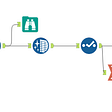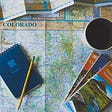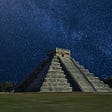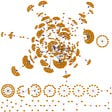# Geospatial Workflows to Estimate a City’s Population

How many people are there per square mile of New York? How can the number of people in a locality be identified during a hurricane? Enter Geospatial Analytics, which uses a combination of satellite images and data analysis.

Geospatial AI and Spatial Data Analysis have a more visual flavor to it than numbers. The output is visual, but there are several steps involved to get there. This blog will look at how to approach a geospatial problem as a data scientist, where one would take a step by step approach to wrangling and visualizing the data to arrive at the result.

This analysis of the processing pipeline is good for two reasons. It gives you a better understanding of your data and makes tracking bugs easier. Without further ado, let’s take up the problem of finding out where people live in a city.

We can get population data at a low spatial and temporal resolution. Censuses are usually the go-to resource for this kind of data. But in most countries, the census is a decennial exercise that can give us a granularity of ward level (best case scenario).

Governments and Nonprofits design policies and interventions based on these outdated data. In the event of an epidemic or natural disaster, it would be better to know exactly where people live and track their movements.

So how can you get updated data? Let’s try to solve the problem using spatial data science to estimate the human population at a 100x100 meter grid level. We can use building footprints as a proxy for human settlements and use a global data source like the Gridded Population of the World (GPW) to estimate the population at such a granular resolution.

Where do people live? Let’s find out with Geospatial Analytics.

Let’s get started by choosing a city. For this example, we focus on the tourism city of Uganda, Fort Portal.

`REGION = ‘fortportal’UTM = 32636PIPELINE = ‘output’`

Now, let’s look at the steps to achieve this.

# Load GeoJSON and Population Data

The first step is to load the city’s boundaries with GeoJSON.

`region = gpd.read_file(INPUT/f’{REGION}.geojson’).to_crs(epsg=WGS84)box = region.total_boundsshow(region)`

• Read the Gridded Population of the World (GPW) dataset, which is available as a Raster TIFF file
• Clip it to the city boundary using the GeoJSON file
• Save the clipped raster
`gpw = rio.open(INPUT/’gpw_world.tif’)gpw_region = gpw.read(1, window=gpw.window(*box))# Clip to the region boundaryregion_mask, region_mask_tfm = mask(dataset=gpw, shapes=region.geometry, all_touched=True, crop=True, filled=True)region_mask = np.where(region_mask < 0, 0, region_mask).squeeze()# Save the clipped rasterregion_meta = gpw.metaregion_meta.update(dict(driver=’GTiff’,height=region_mask.shape,width=region_mask.shape,transform=region_mask_tfm))with rio.open(INTER/f’{REGION}_gpw_output.tif’, ‘w’, **region_meta) as f: f.write(region_mask, indexes=1)show(region, region_mask)`

# 2. Polygonize

Population data for Fort Portal is now available as a raster TIFF file. To read it as a GeoDataFrame and perform spatial operations, we need to polygonize this raster into a vector format.

We can do this using the rasterio library in python.

Pro tip: Use rasterio CLI inside Jupyter notebooks and save a few lines of code

`!rio shapes {INTER/REGION}_gpw_output.tif — bidx 1 — precision 6 > {INTER/REGION}_gpw_output.geojson# of tiles: 68, Area covered: 61.96 km.sqPopulation statistics: mean: 1068.56, median: 1025.65, std: 547.29`

# 3. Gridify

The vectorized GPW GeoJSON file gives us the city’s population at a 1km x 1km spatial resolution. But we intend to estimate the population at 100x100m spatial resolution. To achieve this, we have to:

• Gridify: Split the 1km x 1km tile into smaller grids of size 100m x 100m
• Each 1km x 1km tile will give us 100 grids of size 100m x 100m

Pro tip: Gridification works well only for the MERCATOR projection. Ensure that your GeoData Frames have proper CRS before performing this operation.

`def gridify(tile):polygons = []xmin,ymin,xmax,ymax = tile.geometry.boundswidth = tile.tile_widthheight = tile.tile_heightstepx = +(xmax — xmin)/(10 * width )stepy = -(ymax — ymin)/(10 * height)for x in np.arange(xmin, xmax, stepx):for y in np.arange(ymax, ymin, stepy):poly = [(x , y ),(x + stepx, y ),(x + stepx, y + stepy),(x , y + stepy)]polygons.append(Polygon(poly))d = {‘geometry’: polygons,‘tile_idx’: tile.tile_idx,‘tile_population’: tile.tile_population,‘tile_width’: tile.tile_width,‘tile_height’: tile.tile_height}grids_gdf = gpd.GeoDataFrame(d, crs=f’EPSG:{MERCATOR}’)tile_gdf = gpd.GeoDataFrame(tile.to_frame().T, crs=f’EPSG:{MERCATOR}’)grids_gdf = gpd.clip(grids_gdf, tile_gdf)return grids_gdf# For each TILE create the GRIDsgrids_gdf = tiles_gdf.apply(gridify, axis=1)grids_gdf = pd.concat(grids_gdf.to_list())grids_gdf = grids_gdf.reset_index(drop=True).reset_index().rename(columns={‘index’: ‘idx’}).to_crs(epsg=UTM)# Change the CRS of TILEs & GRIDs back to their region respective UTM coordinatestiles_gdf = tiles_gdf.to_crs(epsg=UTM)grids_gdf = grids_gdf.to_crs(epsg=UTM)region = region.to_crs(epsg=UTM)grids_gdf.head()`

# 4. Load Building Footprints Dataset

In this example, we use buildings as a proxy for estimating the human population.

Luckily for us, Microsoft has released building footprints for multiple regions around the world. The building footprints for Fort Portal in Uganda are also available.

If the region you’re considering doesn’t have readily available building footprints, you can try these alternatives:

• Check Open Street Maps (OSM). They have good coverage in several places worldwide. Also, consider contributing to it
• Put your deep learning skills to the test. Try getting your hands on some satellite imagery of the region and generate building footprints using segmentation models. Dave Luo has written a nice article about segmenting buildings with geodata tools.
`footprints_gdf = (gpd.read_file(f’{INPUT/REGION}_footprints.geojson’).to_crs(epsg=UTM).assign(centroid=lambda x: x.centroid,building_area=lambda x:x.geometry.area).rename(columns={‘geometry’: ‘building_count’}).set_geometry(‘centroid’))footprints_gdf.head()`

# 5. Compute Building Statistics for Each Grid

Now, to support this geospatial workflow, we have the following:

• Grids at 100m x 100m resolution
• Building footprints for the city

We would like to find the buildings falling under each grid and then compute how many are there and their occupancy rates. We can do a spatial join between the two Data Frames, which is much faster thanks to Geopandas (version > 0.8). We take the centroid of each building’s footprint and do a spatial join with the grid boundaries.

`def sjoin_polygon_footprints(poly_gdf, footprints_gdf, idx, name, agg):poly_gdf = gpd.sjoin(poly_gdf, footprints_gdf, how=’left’, op=’intersects’)poly_gdf = (poly_gdf.groupby(idx).agg(agg).reset_index())poly_gdf = (gpd.GeoDataFrame(poly_gdf, crs=f’EPSG:{UTM}’).rename(columns={‘building_area’ : f’{name}_building_area’,‘building_count’: f’{name}_building_count’}))return poly_gdfagg = {‘geometry’ : ‘first’,‘building_area’ : ‘sum’,‘building_count’ : ‘count’,‘tile_idx’ : ‘first’,‘tile_population’ : ‘first’,‘tile_width’ : ‘first’,‘tile_height’ : ‘first’}grids_gdf = sjoin_polygon_footprints(grids_gdf, footprints_gdf, ‘idx’, ‘grid’, agg=agg)grids_gdf.head()`

# 6. Remove Extra Grids, Adjust Population

The GPW population data is available to us at 1km x 1km resolution. We are clipping that to the region boundary.

When we split the tile into grids, along the edges there will be grids that fall beyond the region boundary. However, in this geospatial workflow, we will not have building footprints available for areas outside our region boundary. So, we remove the extra grids and adjust the population count by taking a ratio of the number of grids that fall within the region boundary.

`grids_gdf = gpd.sjoin(grids_gdf, region[[‘geometry’]], how=’inner’, op=’intersects’).drop(labels=’index_right’, axis=1)# Fix the index of the gridsgrids_gdf = (grids_gdf.drop(labels=’idx’, axis=1).reset_index(drop=True).reset_index().rename(columns={‘index’: ‘idx’}))# Adjust the population accordinglydef recompute_population_by_grids(gp):if gp.grid_building_count.sum() <= 1: gp.tile_population = 0return gp.tile_population * (gp.shape / (gp.tile_width * gp.tile_height * 100))grids_gdf[‘tile_population’] = (grids_gdf.groupby(‘tile_idx’).apply(recompute_population_by_grids).values)show(region, grids_gdf)`

# 7. Compute the Population at the Grid Level

For each grid, we now have the tile population, the number of buildings inside its boundary, and the area of buildings within the boundary.

We can statistically distribute the tile population to the grids by considering the building properties, as buildings prove to be good proxies for this.

`# Tile population distributed by the ratio of # of buildings for each gridgrid_population_count = tile_population * (grid_building_count / tile_building_count)# Tile population distributed by the ratio of area of buildings for each gridgrid_population_area = tile_population * (grid_building_area / tile_building_area )grid_population = Weighted average of the above 2 metricsgrids_gdf[[‘tile_building_count’, ‘tile_building_area’]] = grids_gdf.groupby(‘tile_idx’)[[‘grid_building_count’, ‘grid_building_area’]].transform(‘sum’)# Statistically distribute the TILE population to GRIDsgrids_gdf[‘grid_population’] = (0.5 * grids_gdf[‘tile_population’] * (grids_gdf[‘grid_building_count’] / grids_gdf[‘tile_building_count’]) +0.5 * grids_gdf[‘tile_population’] * (grids_gdf[‘grid_building_area’ ] / grids_gdf[‘tile_building_area’ ]))grids_gdf.loc[:, ‘grid_population’] = grids_gdf[‘grid_population’].fillna(0)grids_gdf.head()`

Pro tip: If you would like to improve this model’s accuracy, consider using DEM and DSM datasets to find building height and use this as a factor to distribute the population. More the number of floors, the higher the population.

# The population at various granularities

Let’s take a look at how the population varies at the 1x1 km and 100x100 m resolutions.

Pro tip: If you need to process the data further, consider using the “feather” format available in Geopandas, as it speeds up the reading and writing of GeoJSON files.

Now, let’s save this:

`grids_gdf.to_crs(WGS84).to_file(f’{OUTPUT/REGION}_grids_output_{WGS84}.geojson’, driver=’GeoJSON’)`

Hopefully, this gives you a fair idea of why and how to use Jupyter Notebooks to create Geospatial workflows.

## More from Gramener

Gramener is a design-led data science company that solves complex business problems with compelling data stories using insights and a low-code platform, Gramex.

Love podcasts or audiobooks? Learn on the go with our new app.

## Create an Analytical Dataset## Data and Data Pipelines## Generate interactive maps using Folium## Big Data and Central Banks.## Beer Recommendations using Collaborative Filtering with Neo4j## Predicting COVID-19 Intensive Zones in Delhi using Neighborhood Clustering## Why VR? The Many Benefits of Immersive Data Analysis## How to deal categorical variables in predictive analytics.## Gramener

Gramener is a design-led data science company that solves complex business problems with compelling data stories using insights and a low-code platform, Gramex.

## When satellite images look into the past: Earth observation & archaeology## How I built a wind map with MTS## Make Your Graphs Available For Visualization## How To Effectively Track Ships And Find Vessels From Space Using AIS And Satellite Imageries?